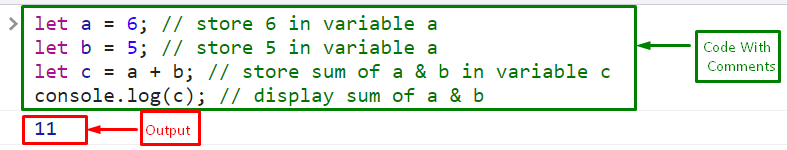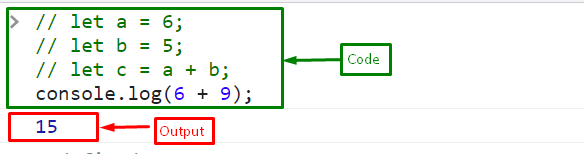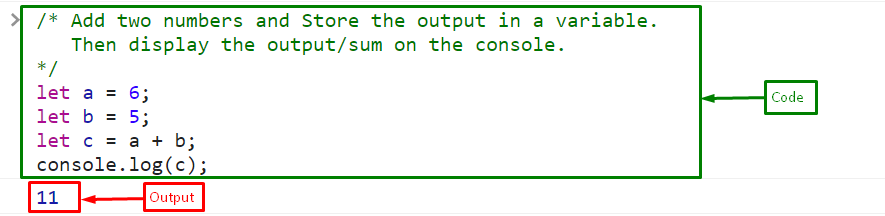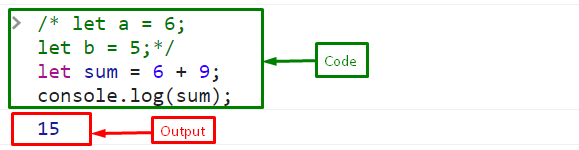# How to Comment Out Multiple Lines in JavaScript

While writing code in JavaScript, it’s common to add comments to explain the purpose or functionality of certain sections or code. Sometimes, developers comment out the specific line of code for hiding some functionality for a particular time period. More specifically, comments not only improve code readability but also make it easy and simple for other programmers to understand your code.

This blog will define the method to comment out the multiple lines in JavaScript.

## How to Comment Out Multiple Lines in JavaScript?

There are two ways for including comments in JavaScript code:

• Inline comment syntax
• Block comment syntax

## Method 1: Comment Out Multiple Lines Using “Inline Comment Syntax”

Inline comment syntax” is also known as “single line comment” as it is mostly used for commenting on single lines or for explaining code. It is also utilized for commenting multiple lines in JavaScript but for each line adding this comment syntax is a little bit hard. So, for this developers select the specific section for commenting and use the shortcut key “ctrl+/”.

### Syntax

For commenting on single or multiple lines in JavaScript, the following syntax is used for Inline comments:

`// something`

Each line starting with “//” is considered a comment and will not be interpreted/executed by JavaScript.

### Example

In the given example, we will add two numbers and display them on the console and each line of code is explained with the help of comments. It helps other programmers to understand the code that how it works:

```let a = 6; // store 6 in variable a
let b = 5; // store 5 in variable a
let c = a + b; // store sum of a & b in variable c
console.log(c); // display sum of a & b```

As you can see that the commented lines will not execute and the output only displayed the sum of two numbers:Here, we will comment out multiple lines of code using the inline comment syntax. Comment out the first three lines of code which contain variables “a”, “b” and “c” that store the numbers and their sum. Then, display the sum of two numbers “6” and “9” using the “console.log()” method:

```// let a = 6;
// let b = 5;

// let c = a + b;
console.log(6 + 9);```

Output:## Method 2: Comment Out Multiple Lines Using “Block Comment Syntax”

Block comment syntax” is also known as the “multi-line comment” because it is ideal for longer comments or comments that span multiple lines. It is useful to disable code blocks temporarily.

### Syntax

The block comment is added by using the following syntax:

```/* code
code*/```

### Example

First, we will add a block comment to explain the code using the multi-line comment syntax:

```/* Add two numbers and Store the output in a variable.
Then display the output/sum on the console.
*/```

Then, declare two variables “a” and “b” and store two numbers “6” and “5” in it:

```let a = 6;
let b = 5;```

Create another variable “c” that will store the sum of variables “a” and “b”:

`let c = a + b;`

Finally, print the sum on the console using the “console.log()” method:

`console.log(c);`

OutputYou can also comment out the code using the block comment or multi-line comment syntax. Here, we will comment out the variable “a” and “b” and store the sum of “6” and “9” in variable “sum” and then display them on the console:

```/* let a = 6;
let b = 5;*/
let sum = 6 + 9;
console.log(sum);```

OutputThat’s all about commenting multiple lines in JavaScript.

## Conclusion

For commenting on multiple lines in JavaScript, there are two ways: “inline comment” or “multi-line comment”. “Multi-line comment” is also known as the “block comments”. The code or lines between “/* */” is considered as a block comment while for inline comments “//” is used. Each line starting with “//” or in between “/* */” is considered a comment and will not be interpreted/executed by JavaScript. This blog defined the methods for commenting out the multiple lines in JavaScript.